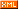# zhuxinyu

posts - 1, comments - 0, trackbacks - 0, articles - 8
::  ::  ::  ::::
8，尽量使用ID代替Class// 创建一个listvar $myList =$('#myList');var myListItems = '
<ul>';for (var i = 0; i
< 1000; i++) {myListItems +
= '<li class="listItem' + i + '">This is a list item</li>'; //这里使用的是class}myListItems += '
</ul>';$myList.html(myListItems);// 选择每一个 lifor (var i = 0; i < 1000; i++) {var selectedItem =$('.listItem' + i);}// 创建一个listvar $myList =$('#myList');var myListItems = '
<ul>';for (var i = 0; i
< 1000; i++) {myListItems +
= '<li id="listItem' + i + '">This is a list item</li>'; //这里使用的是id}myListItems += '
</ul>';$myList.html(myListItems);// 选择每一个 lifor (var i = 0; i < 1000; i++) {var selectedItem =$('#listItem' + i);}

9，给选择器一个上下文

jQuery选择器中有一个这样的选择器，它能指定上下文。
jQuery( expression, context );$("p").click(function() {alert($(this).text() );});$("button").click(function() {$("
<p>this is second p</p>").click(function(){  //为新增的元素重新绑定一次alert( $(this).text() );}).appendTo("body");});}) 虽然我把绑定事件重新写了一次，代码多了点，但这种方式的效率明显高于live()方式， 特别是在频繁的DOM操作中，这点非常明显。 11，子选择器和后代选择器 后代选择器经常用到，比如：$("#list  p");$("button").click(function(){if($("p").data("flag")){$("p").text("true");$("p").data("flag",false);}else{$("p").text("false");$("p").data("flag",true);}});})

13，尽量使用原生的JavaScript方法$(document).ready(function(){var$cr = $("#cr"); //jQuery对象$cr.click(function(){if($cr.is(":checked")){ //jQuery方式判断alert("感谢你的支持!你可以继续操作！");}})}); 上面代码中，判断是否选中是用了 jquery的方法，但这里可以直接使用原生的JavaScript方法，看下面代码：Code 毋庸置疑，第二种方式效率高于第一种方式，因为他不需要拐弯抹角的去调用许多函数。 更多：$(this).css("color","red");   ...... 改成： this.style.color ="red"   ......

$("<p></p>") 改成:$( document.createElement("p") )

14，使用for代替each()方法var array = new Array ();for (var i=0; i
<10000; i++) {array[i]
= 0;}$.each (array, function (i) {array[i] = i;}); 15，使用join()来拼接字符串。 也许你之前一直使用 " + " 来 拼接长字符串。现在你可以改改了。虽然它可能会有点奇怪，但它确实有助于优化性能，尤其是长字符串处理的时候。 首先创建一个数组，然后循环，最后使用join()把数组转化为字符串 var array = []; for (var i=0; i <=10000; i++) { array[i] = '<li>'+i+'</li>'; }$('#list').html (array.join (''));

16，Ajax中文乱码。
Ajax传递中文时会乱码，传统方式大家都是利用escape去编码，然后去后台解码。$.ajax({type: "POST",url: "AjaxTest.jsp",data: "txt="+$('#txt').val(),success: function(msg) {$("#AjaxResponse").text(msg);}}); 现在你可以换一换了，你可以非常简单的解决这个问题，只需要添加一参数即可：$.ajax({type: "POST",url: "AjaxTest.jsp",data: "txt="+$('#tbox1').val(),success: function(msg){$("#AjaxResponse").text(msg);},contentType:"application/x-www-form-urlencoded;charset=utf-8"});